# 高階函數## Curried functions

ghci> max 4 55ghci> (max 4) 55multThree :: (Num a) => a -> a -> a -> amultThree x y z = x * y * z

ghci> let multTwoWithNine = multThree 9ghci> multTwoWithNine 2 354ghci> let multWithEighteen = multTwoWithNine 2ghci> multWithEighteen 10180

compareWithHundred :: (Num a, Ord a) => a -> OrderingcompareWithHundred x = compare 100 x

compareWithHundred :: (Num a, Ord a) => a -> OrderingcompareWithHundred = compare 100

Yo! 你得保證已經弄明白了 Curried functions 與不全呼叫的原理，它們很重要！

divideByTen :: (Floating a) => a -> adivideByTen = (/10)

isUpperAlphanum :: Char -> BoolisUpperAlphanum = (elem ['A'..'Z'])

ghci> multThree 3 4:1:0:No instance for (Show (t -> t))arising from a use of print' at :1:0-12Possible fix: add an instance declaration for (Show (t -> t))In the expression: print itIn a 'do' expression: print it

ghci 說，這一表達式回傳了一個 a -> a 型別的函數，但它不知道該如何顯示它。 函數不是 Show 型別類的實例，所以我們不能得到表示一函數內容的字串。 若在 ghci 中計算 1+1，它會首先計算得 2，然後呼叫 show 2 得到該數值的字串表示，即 "2"，再輸出到屏幕.

## 是時候了，來點高階函數！

applyTwice :: (a -> a) -> a -> a  applyTwice f x = f (f x)*Note*: 現在開始我們會直說某函數含有多個參數(除非它真的只有一個參數)。 以簡潔之名，我們會說 (a->a->a) 取兩個參數，儘管我們知道它在背後做的手腳.

ghci> applyTwice (+3) 10  16  ghci> applyTwice (++ " HAHA") "HEY"  "HEY HAHA HAHA"  ghci> applyTwice ("HAHA " ++) "HEY"  "HAHA HAHA HEY"  ghci> applyTwice (multThree 2 2) 9  144  ghci> applyTwice (3:)   [3,3,1]

zipWith' :: (a -> b -> c) -> [a] -> [b] -> [c]  zipWith' _ [] _ = []  zipWith' _ _ [] = []  zipWith' f (x:xs) (y:ys) = f x y : zipWith' f xs ys

ghci> zipWith' (+) [4,2,5,6] [2,6,2,3]  [6,8,7,9]  ghci> zipWith' max [6,3,2,1] [7,3,1,5]  [7,3,2,5]  ghci> zipWith' (++) ["foo "，"bar "，"baz "] ["fighters"，"hoppers"，"aldrin"]  ["foo fighters","bar hoppers","baz aldrin"]  ghci> zipWith' (*) (replicate 5 2) [1..]  [2,4,6,8,10]  ghci> zipWith' (zipWith' (*)) [[1,2,3],[3,5,6],[2,3,4]] [[3,2,2],[3,4,5],[5,4,3]]  [[3,4,6],[9,20,30],[10,12,12]]

flip' :: (a -> b -> c) -> (b -> a -> c)  flip' f = g      where g x y = f y x

flip' :: (a -> b -> c) -> b -> a -> c  flip' f y x = f x y

ghci> flip' zip [1,2,3,4,5] "hello"  [('h',1),('e',2),('l',3),('l',4),('o',5)]  ghci> zipWith (flip' div) [2,2..] [10,8,6,4,2]  [5,4,3,2,1]

## map 與 filter

map 取一個函數和 List 做參數，遍歷該 List 的每個元素來呼叫該函數產生一個新的 List。 看下它的型別聲明和實現:

map :: (a -> b) -> [a] -> [b]  map _ [] = []  map f (x:xs) = f x : map f xs

ghci> map (+3) [1,5,3,1,6]  [4,8,6,4,9]  ghci> map (++ "!") ["BIFF"，"BANG"，"POW"]  ["BIFF!","BANG!","POW!"]  ghci> map (replicate 3) [3..6]  [[3,3,3],[4,4,4],[5,5,5],[6,6,6]]  ghci> map (map (^2)) [[1,2],[3,4,5,6],[7,8]]  [[1,4],[9,16,25,36],[49,64]]  ghci> map fst [(1,2),(3,5),(6,3),(2,6),(2,5)]  [1,3,6,2,2]

filter 函數取一個限制條件和一個 List，回傳該 List 中所有符合該條件的元素。它的型別聲明及實現大致如下:

filter :: (a -> Bool) -> [a] -> [a]  filter _ [] = []  filter p (x:xs)       | p x       = x : filter p xs      | otherwise = filter p xs

ghci> filter (>3) [1,5,3,2,1,6,4,3,2,1]  [5,6,4]  ghci> filter (==3) [1,2,3,4,5]    ghci> filter even [1..10]  [2,4,6,8,10]  ghci> let notNull x = not (null x) in filter notNull [[1,2,3],[],[3,4,5],[2,2],[],[],[]]  [[1,2,3],[3,4,5],[2,2]]  ghci> filter (elem ['a'..'z']) "u LaUgH aT mE BeCaUsE I aM diFfeRent"  "uagameasadifeent"  ghci> filter (elem ['A'..'Z']) "i lauGh At You BecAuse u r aLL the Same"  "GAYBALLS"

quicksort :: (Ord a) => [a] -> [a]    quicksort [] = []    quicksort (x:xs) =         let smallerSorted = quicksort (filter (<=x) xs)        biggerSorted = quicksort (filter (>x) xs)       in  smallerSorted ++ [x] ++ biggerSorted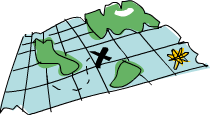mapfilter 是每個函數式程序員的麵包黃油(呃，mapfilter 還是 List Comprehension 並不重要)。 想想前面我們如何解決給定周長尋找合適直角三角形的問題的? 在命令式編程中，我們可以套上三個循環逐個測試當前的組合是否滿足條件，若滿足，就打印到屏幕或其他類似的輸出。 而在函數式編程中，這行就都交給 mapfilter。 你弄個取一參數的函數，把它交給 map 過一遍 List，再 filter 之找到合適的結果。 感謝 Haskell 的惰性，即便是你多次 map 一個 List 也只會遍歷一遍該 List，要找出小於 100000 的數中最大的 3829 的倍數，只需過濾結果所在的 List 就行了.

largestDivisible :: (Integral a) => a  largestDivisible = head (filter p [100000,99999..])      where p x = x mod 3829 == 0

ghci> sum (takeWhile (<10000) (filter odd (map (^2) [1..])))  166650

ghci> sum (takeWhile (<10000) [m | m <- [n^2 | n <- [1..]], odd m])  166650

chain :: (Integral a) => a -> [a]  chain 1 =   chain n      | even n =  n:chain (n div 2)      | odd n  =  n:chain (n*3 + 1)

ghci> chain 10  [10,5,16,8,4,2,1]  ghci> chain 1    ghci> chain 30  [30,15,46,23,70,35,106,53,160,80,40,20,10,5,16,8,4,2,1]

yay! 貌似工作良好。 現在由這個函數來告訴我們結果:

numLongChains :: Int  numLongChains = length (filter isLong (map chain [1..100]))      where isLong xs = length xs > 15

*Note*: 這函數的型別為 numLongChains :: Int。這是由於歷史原因，length 回傳一個 Int 而非 Num 的成員型別，若要得到一個更通用的 Num a，我們可以使用 fromIntegral 函數來處理所得結果.

map，我們可以寫出類似 map (*) [0..] 之類的程式碼。 如果只是為了例證 Curried functions 和不全呼叫的函數是真正的值及其原理，那就是你可以把函數傳遞或把函數裝在 List 中(只是你還不能將它們轉換為字串)。 迄今為止，我們還只是 map 單參數的函數到 List，如 map (*2) [0..] 可得一組型別為 (Num a) => [a] 的 List，而 map (*) [0..] 也是完全沒問題的。* 的型別為 (Num a) => a -> a -> a，用單個參數呼叫二元函數會回傳一個一元函數。如果用 *map 一個 [0..] 的 List，就會得到一組一元函數組成的 List，即 (Num a) => [a->a]map (*) [0..] 所得的結果寫起來大約就是 [(0*),(1*),(2*)..].

ghci> let listOfFuns = map (*) [0..]  ghci> (listOfFuns !! 4) 5  20

## lambda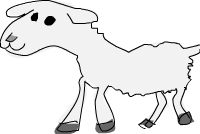lambda 就是匿名函數。有些時候我們需要傳給高階函數一個函數，而這函數我們只會用這一次，這就弄個特定功能的 lambda。編寫 lambda，就寫個 \ (因為它看起來像是希臘字母的 lambda — 如果你斜視的厲害)，後面是用空格分隔的參數，-> 後面就是函數體。通常我們都是用括號將其括起，要不然它就會佔據整個右邊部分。

numLongChains :: Int  numLongChains = length (filter (\xs -> length xs > 15) (map chain [1..100]))lambda 是個表達式，因此我們可以任意傳遞。表達式 (\xs -> length xs > 15) 回傳一個函數，它可以告訴我們一個 List 的長度是否大於 15。

ghci> zipWith (\a b -> (a * 30 + 3) / b) [5,4,3,2,1] [1,2,3,4,5]  [153.0,61.5,31.0,15.75,6.6]

ghci> map (\(a,b) -> a + b) [(1,2),(3,5),(6,3),(2,6),(2,5)]  [3,8,9,8,7]

addThree :: (Num a) => a -> a -> a -> a  addThree x y z = x + y + z
addThree :: (Num a) => a -> a -> a -> a  addThree = \x -> \y -> \z -> x + y + z

flip' :: (a -> b -> c) -> b -> a -> c  flip' f = \x y -> f y x

## 關鍵字 fold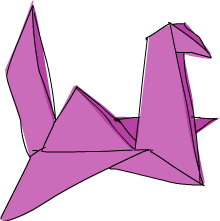sum' :: (Num a) => [a] -> a  sum' xs = foldl (\acc x -> acc + x) 0 xs

ghci> sum' [3,5,2,1]  11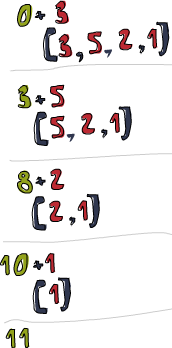sum' :: (Num a) => [a] -> a  sum' = foldl (+) 0

elem' :: (Eq a) => a -> [a] -> Bool  elem' y ys = foldl (\acc x -> if x == y then True else acc) False ys

map' :: (a -> b) -> [a] -> [b]  map' f xs = foldr (\x acc -> f x : acc) [] xs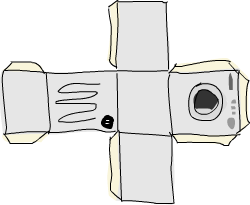foldl1foldr1 的行為與 foldlfoldr 相似，只是你無需明確提供初始值。他們假定 List 的首個(或末尾)元素作為起始值，並從旁邊的元素開始摺疊。這一來，sum 函數大可這樣實現：sum = foldl1 (+)。這裡待摺疊的 List 中至少要有一個元素，若使用空 List 就會產生一個運行時錯誤。不過 foldlfoldr 與空 List 相處的就很好。所以在使用 fold 前，應該先想下它會不會遇到空 List，如果不會遇到，大可放心使用 foldr1foldl1

maximum' :: (Ord a) => [a] -> a  maximum' = foldr1 (\x acc -> if x > acc then x else acc)  reverse' :: [a] -> [a]  reverse' = foldl (\acc x -> x : acc) []  product' :: (Num a) => [a] -> a  product' = foldr1 (*)  filter' :: (a -> Bool) -> [a] -> [a]  filter' p = foldr (\x acc -> if p x then x : acc else acc) []  head' :: [a] -> a  head' = foldr1 (\x _ -> x)  last' :: [a] -> a  last' = foldl1 (\_ x -> x)

scanlscanrfoldlfoldr 相似，只是它們會記錄下累加值的所有狀態到一個 List。也有 scanl1scanr1

ghci> scanl (+) 0 [3,5,2,1]  [0,3,8,10,11]  ghci> scanr (+) 0 [3,5,2,1]  [11,8,3,1,0]  ghci> scanl1 (\acc x -> if x > acc then x else acc) [3,4,5,3,7,9,2,1]  [3,4,5,5,7,9,9,9]  ghci> scanl (flip (:)) [] [3,2,1]  [[],,[2,3],[1,2,3]]

sqrtSums :: Int  sqrtSums = length (takeWhile (<1000) (scanl1 (+) (map sqrt [1..]))) + 1
ghci> sqrtSums  131  ghci> sum (map sqrt [1..131])  1005.0942035344083  ghci> sum (map sqrt [1..130])  993.6486803921487

scan 可以用來跟蹤 fold 函數的執行過程。想想這個問題，取所有自然數的平方根的和，尋找在何處超過 1000？ 先map sqrt [1..]，然後用個 fold 來求它們的和。但在這裡我們想知道求和的過程，所以使用 scanscan 完畢時就可以得到小於 1000 的所有和。所得結果 List 的第一個元素為 1，第二個就是 1+根2，第三個就是 1+根2+根3。若有 x 個和小於 1000，那結果就是 x+1

## 有$的函數呼叫 好的，接下來看看$ 函數。它也叫作函數呼叫符。先看下它的定義：

($) :: (a -> b) -> a -> b f$ x = f xsum (filter (> 10) (map (*2) [2..10])) 該如何？嗯，$ 是右結合，f (g (z x))f$ g $z x 等價。所以我麼可以將 sum (filter (> 10) (map (*2) [2..10]) 重寫為 sum$ filter (> 10) $map (*2) [2..10] 除了減少括號外，$ 還可以將數據作為函數使用。例如映射一個函數呼叫符到一組函數組成的 List：

ghci> map ($3) [(4+),(10*),(^2),sqrt] [7.0,30.0,9.0,1.7320508075688772] ## Function composition 在數學中，函數組合是這樣定義的：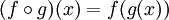，表示組合兩個函數成為一個函數。以 x 呼叫這一函數，就與用 x 呼叫 g 再用所得的結果呼叫 f 等價。 Haskell 中的函數組合與之很像，即 . 函數。其定義為： (.) :: (b -> c) -> (a -> b) -> a -> c f . g = \x -> f (g x)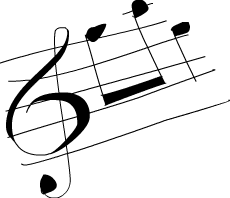注意下這型別聲明，f 的參數型別必須與 g 的回傳型別相同。所以得到的組合函數的參數型別與 g 相同，回傳型別與 f 相同。表達式 negate . (*3) 回傳一個求一數字乘以 3 後的負數的函數。 函數組合的用處之一就是生成新函數，並傳遞給其它函數。當然我們可以用 lambda 實現，但大多數情況下，使用函數組合無疑更清楚。假設我們有一組由數字組成的 List，要將其全部轉為負數，很容易就想到應先取其絶對值，再取負數，像這樣： ghci> map (\x -> negate (abs x)) [5,-3,-6,7,-3,2,-19,24] [-5,-3,-6,-7,-3,-2,-19,-24] 注意下這個 lambda 與那函數組合是多麼的相像。用函數組合，我們可以將程式碼改為： ghci> map (negate . abs) [5,-3,-6,7,-3,2,-19,24] [-5,-3,-6,-7,-3,-2,-19,-24] 漂亮！函數組合是右結合的，我們同時組合多個函數。表達式 f (g (z x))(f . g . z) x 等價。按照這個思路，我們可以將 ghci> map (\xs -> negate (sum (tail xs))) [[1..5],[3..6],[1..7]] [-14,-15,-27] 改為： ghci> map (negate . sum . tail) [[1..5],[3..6],[1..7]] [-14,-15,-27] 不過含多個參數的函數該怎麼辦？好，我們可以使用不全呼叫使每個函數都只剩下一個參數。sum (replicate 5 (max 6.7 8.9)) 可以重寫為 (sum . replicate 5 . max 6.7) 8.9sum . replicate 5 . max 6.7$ 8.9。在這裡會產生一個函數，它取與 max 6.7 同樣的參數，並使用結果呼叫 replicate 5 再用 sum 求和。最後用 8.9 呼叫該函數。不過一般你可以這麼讀，用 8.9 呼叫 max 6.7，然後使它 replicate 5，再 sum 之。如果你打算用函數組合來替掉那堆括號，可以先在最靠近參數的函數後面加一個 $，接着就用 . 組合其所有函數呼叫，而不用管最後那個參數。如果有這樣一段程式碼：replicate 100 (product (map (*3) (zipWith max [1,2,3,4,5] [4,5,6,7,8])))，可以改為：replicate 100 . product . map (*3) . zipWith max [1,2,3,4,5]$ [4,5,6,7,8]。如果表達式以 3 個括號結尾，就表示你可以將其修改為函數組合的形式。

sum' :: (Num a) => [a] -> a     sum' xs = foldl (+) 0 xs

fn x = ceiling (negate (tan (cos (max 50 x))))

fn = ceiling . negate . tan . cos . max 50

mapfilter 那節中，我們求了小於 10000 的所有奇數的平方的和。如下就是將其置於一個函數中的樣子：

oddSquareSum :: Integer  oddSquareSum = sum (takeWhile (<10000) (filter odd (map (^2) [1..])))

oddSquareSum :: Integer  oddSquareSum = sum . takeWhile (<10000) . filter odd . map (^2) $[1..] 不過若是給別人看，我可能就這麼寫了： oddSquareSum :: Integer oddSquareSum =  let oddSquares = filter odd$ map (^2) [1..]          belowLimit = takeWhile (<10000) oddSquares      in  sum belowLimit